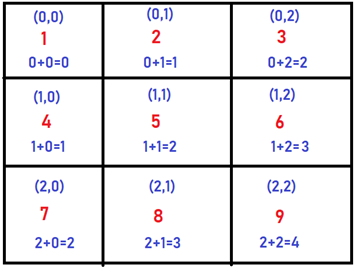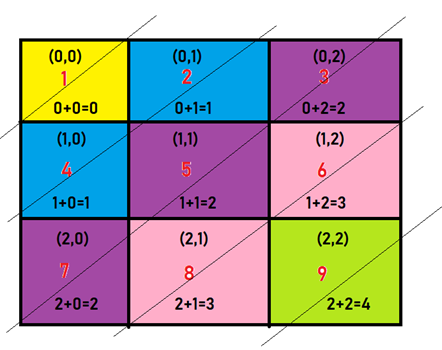# Diagonal Traverse ii

## Introduction

Diagonal traversal is a traversal-based problem where we have to traverse the list of integers diagonally. It is one of the famously asked problems of Array and Sorting. Concepts related to Array and Sorting should be clear in your head if you have to master Data Structures and Algorithms

In this article, we will discuss different approaches from brute force to an efficient one so that there will be clarity to solve other problems.

Without any ado, let’s move to our problem statement.

## Problem Statement

The problem statement for the Diagonal traversal problem is as follows-

We are given a list of integers, and we have to print the list by diagonally traversing the list. But the trick over here is that the length of all the rows is not the same. So, you cannot do just a simple diagonal traversal.

Let me explain with a quick example for more clarity.

Input: [[1,2,3],[4,5,6],[7,8,9]]

Output: [1,4,2,7,5,3,8,6,9]

As you cannot diagonally do simple traversal, you have to find some patterns.As you can see in a 2D matrix, elements in the same diagonal have the same sum of their indices.

So, if we have all elements with the same sum of their indices together, then it’s just a matter of printing those elements in order.This will be our final solution after calculating the sum of the indices.

Will you be able to solve this problem now?

Try to solve Diagonal traversal II yourself before stepping into the solution.

Let’s move towards our approach.

## Approach 1

In this approach, we will be using HashMap and ArrayDeque. HashMap is used to store key and value pairs, where values should be unique. ArrayDeque provides a way to apply resizable-array in addition to the implementation of the Deque interface.

In this algorithm, we will be using the inbuilt functions of HashMap.

We will be using an extra array for storing the diagonal order traversal elements.

## Code

``````import java.util.ArrayDeque;
import java.util.HashMap;
import java.util.List;
import java.util.Map;

public class Diagonal {
public int[] DiagonalOrder(List<List<Integer>> nums)
{
//diagonal -- element associated with it
Map<Integer, ArrayDeque<Integer>> map= new HashMap<>();

int max= 0, size= 0, index= 0;

//traversing the row in the 2 D array
for(int i= 0; i< nums.size(); i++)
{
//calculating the number of element in the  2D Array
size+= nums.get(i).size();

//traversing inside the row in the 2 D array
for(int j= 0; j< nums.get(i).size(); j++)
{
//diagonal we are currently dealing with
int diagonal= i+j;
//if the diagonal is not present in the HashMap, then creating a entry with that diagonal
map.putIfAbsent(diagonal, new ArrayDeque<Integer>());
//Insertion order is Maintained by ArrayDeque//putting into the Array

//calculating the maximum diagonal at every instant
max= Math.max(max, diagonal);
}
}

//resultant array containing the diagonal order traversal elements
int[] res= new int[size];

//every diagonal is associated with number of elements
for(int i= 0; i<= max; i++)
{
while(!map.get(i).isEmpty())
{
//retiring the elements associated with each diagonal, sequentially
res[index]= map.get(i).poll();
//for moving from one index to another index in the resultant Array
index+= 1;
}
}
return res;
}
}``````

## Analysis of Complexity

Time Complexity: The time complexity of this particular approach will be O(m*n). Where m and n are the sizes of rows and columns, respectively.

Space Complexity: The space complexity will be O(n). An array is needed to store the diagonal array elements.

## Approach 2

We will now discuss an efficient approach to solve this particular problem.

We will be using Stacks to make our solution more efficient. Stack is a linear data that follows LIFO(Last In First Out) order, i.e., the most recently added element to be removed first. You can check out more about Stack Data Structure here

Algorithm

•  For numbers on one diagonal sum of the index of the list and index in the list is the same. This means we know the diagonal of the element when we traverse lists.

• If we swipe from top to bottom from left to right, then elements are traversed in reversed order for each diagonal. This means if we use Stack, then popping from the Stack will give us the correct final order. Another thing - we are traversing diagonals in acs order, so we can add Stack for every diagonal as we go.

This solution is better than the hashmap approach since if you use hashmap, the number of numbers in the matrix is really large, it will cause the hash collision, and then I see them doing the reverse that causes more time.

## Code

``````public int[] DiagonalOrder(List<List<Integer>> nums) {

int count = 0;
List<Stack<Integer>> list = new ArrayList();
for (int i = 0; i < nums.size(); i++) {

List<Integer> oneList = nums.get(i);

for (int j = 0; j < oneList.size(); j++) {
//this is id of the diagonal
int idx = i + j;
//check if we haven't checked this diagonal before
if (list.size() < idx + 1) {
}
list.get(idx).push(oneList.get(j));
++count;
}
}
//now traverse the list of stacks to form the final array
int[] res = new int[count];
int p = 0;

for (Stack<Integer> stack : list) {
while(!stack.isEmpty()) {
res[p++] = stack.pop();
}
}
return res;
}``````

## Analysis of Complexity

Time Complexity: O(n) time as we traverse every element of the list once; we do constant work for each element. Then we again traverse every element once to form the resulting array.

Space Complexity: O(n) space as we need to put every element of the list to a list of stacks.

1. What do you mean by HashMap?
HashMap allows us to store key and value pairs, where keys should be unique. It is like a Hashtable class but not synchronized. It may have one null key and multiple null values.

2. How does Stack data structure help in coding?
Stack is a linear data structure that performs operations on some order and that order is known as LIFO(Last IN First Out) i.e., the most recently added element is removed first.

3. What do you mean by ArrayDeque?
ArrayDeques have no capacity restrictions. Null elements are prohibited here and it is likely to be faster than Stack when used as a stack.

## Key Takeaways

In this article, we talked about the traversal of lists in a diagonal manner where the length of all rows can be different. We have implemented in Java language along with their time and space complexities with the help of important data structures.

Apart from this, you can view the Data Structures and Algorithms-guided path to start your preparation from scratch.

You can use CodeStudio to practice various DSA questions typically asked in interviews. It will assist you in mastering efficient coding techniques.

Keep Coding!!!